#Function Repository Resource:

# ChernoffFace

Make Chernoff face diagrams

Contributed by: Anton Antonov  |  Anton Antonov
 ResourceFunction["ChernoffFace"][vec] makes a Chernoff face diagram for the numeric vector vec. ResourceFunction["ChernoffFace"][asc] makes a Chernoff face diagram for an Association asc of facial features to numeric values. ResourceFunction["ChernoffFace"][matrix] makes a list of Chernoff face diagrams corresponding to the rows of matrix. ResourceFunction["ChernoffFace"][listOfAssociations] makes a list of Chernoff face diagrams corresponding to the elements of listOfAssociations. ResourceFunction["ChernoffFace"][data,opts] makes Chernoff face diagrams using specified options opts.

## Details and Options

ResourceFunction["ChernoffFace"] makes a Chernoff face diagram for a numeric vector or an association that maps facial features to numbers.
ResourceFunction["ChernoffFace"] works on lists of associations and lists of numeric vectors.
If the argument is a numeric matrix or a list of associations, the columns of the corresponding full array are rescaled first.
ResourceFunction["ChernoffFace"] takes all options of Graphics.
By default ResourceFunction["ChernoffFace"] makes black-and-white diagrams.
ResourceFunction["ChernoffFace"] diagrams are made with 17 face features.
The color function given to the option ColorFunction is used to auto-color the diagram.
The auto-coloring is done by associating colors with the keys "FaceColor", "IrisColor", "NoseColor", "MouthColor" and "EyeBallColor".
Here are the options taken by ResourceFunction["ChernoffFace"]:
 ColorFunction None "MakeSymmetric" Automatic "RescaleRangeFunction" MinMax
Assume that ResourceFunction["ChernoffFace"] is given the argument vec and the specified color function is cf. If the argument vec has only one element, then:
 "FaceColor" cf@vec[]
If the argument vec has two elements, then:
 "FaceColor" cf@vec[] "IrisColor" cf@vec[]
If the argument vec has fewer than seven elements, then:
 "FaceColor" cf@Mean@vec[[1;;2]] "IrisColor" cf@Mean@vec[[3;;-1]]
If the argument vec has more than six elements, then:
 "FaceColor" cf@Mean@vec[[1;;2]] "MouthColor" cf@Mean@vec[[1;;3]] "IrisColor" cf@Mean@vec[[4;;6]] "NoseColor" cf@Mean@vec[[7;;-1]]
In all cases above, the automatic color assigned to "EyeBallColor" is White.

## Examples

### Basic Examples (1)

Here is a Chernoff face diagram with a list of random numbers:

 In:=Out=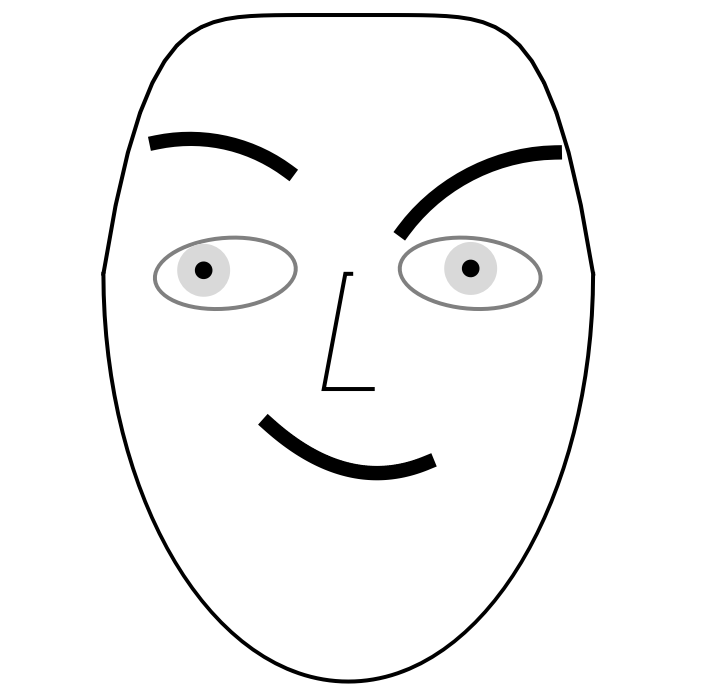### Scope (11)

#### Vector argument (2)

Here is another Chernoff face constructed from random data:

 In:=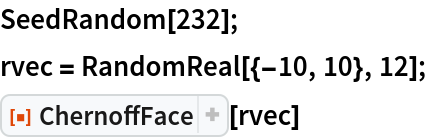Out=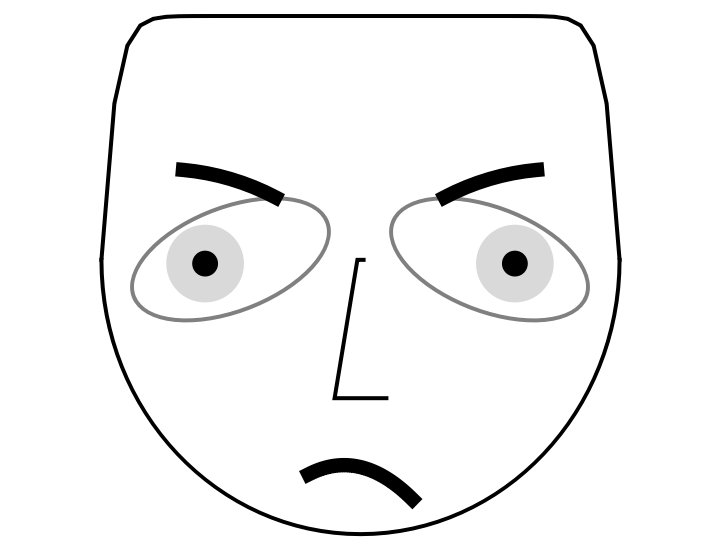If the length of the vector argument is larger than the number of facial features, only the first 17 elements are taken:

 In:=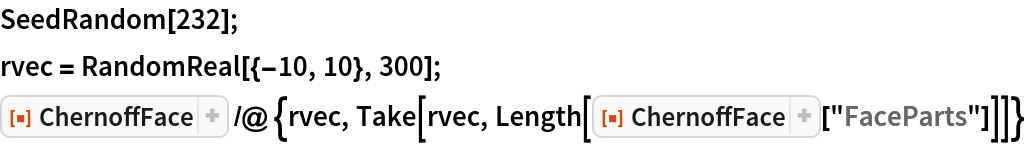Out=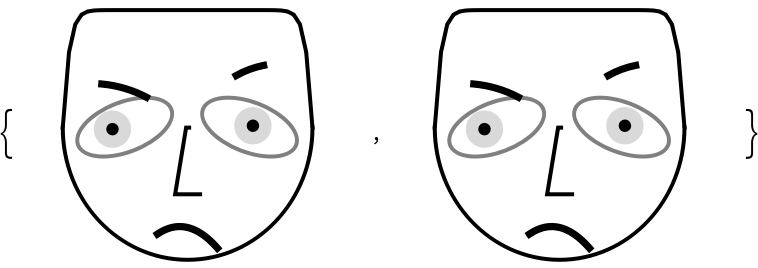In:=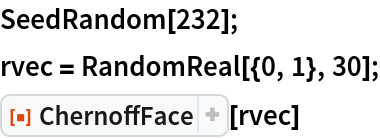Out=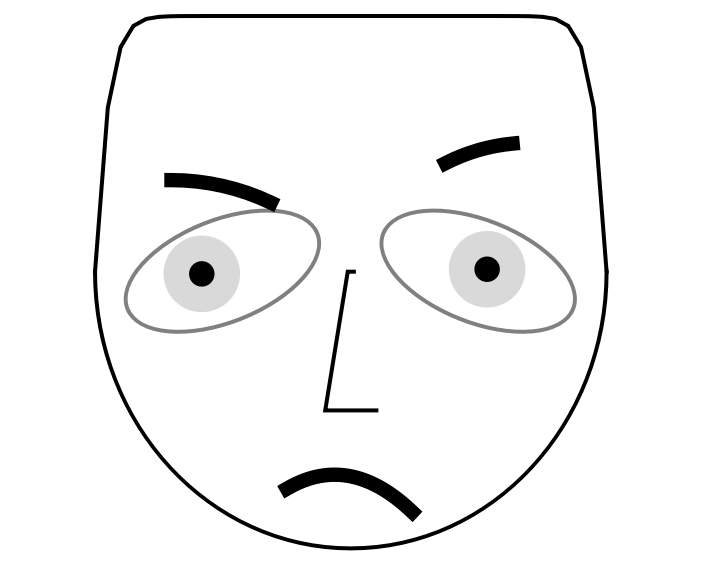#### List of numeric vectors (2)

Here is a random numeric matrix (a list of vectors):

 In:=Visualize the random matrix with Chernoff face diagrams:

 In:=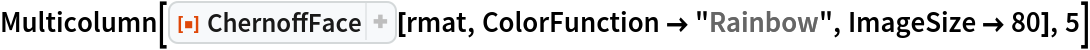Out=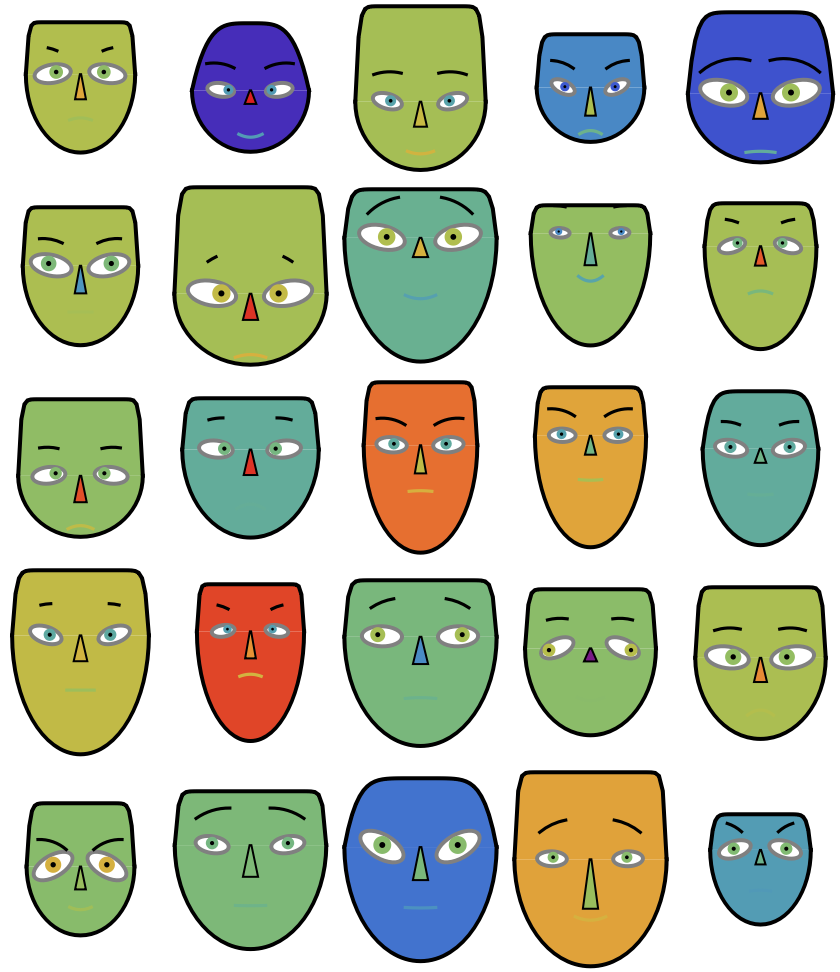#### Summary (1)

Visualize a summary legend that helps the reading of the table of diagrams above:

 In:=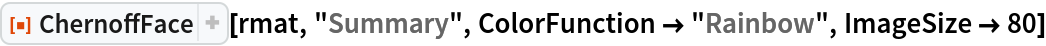Out=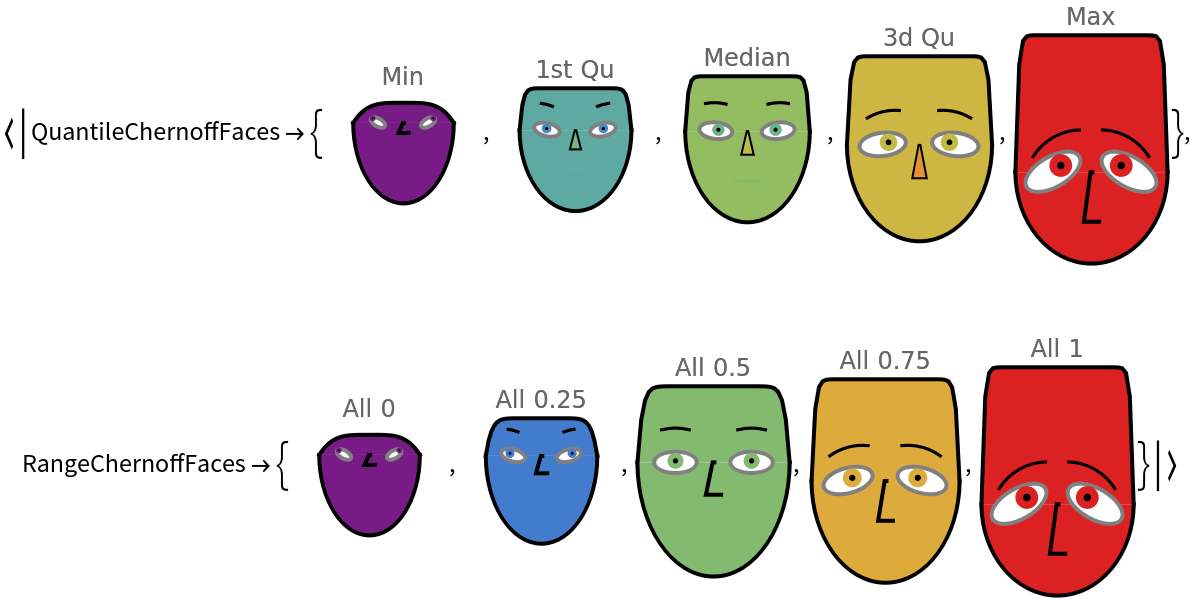#### Association of face parts to values (2)

The main signature of ChernoffFace is using an association that specifies numerical values for different face parts of the diagram:

 In:=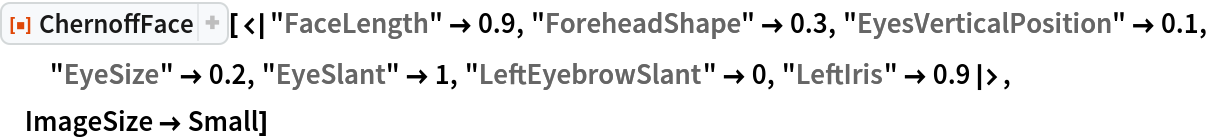Out=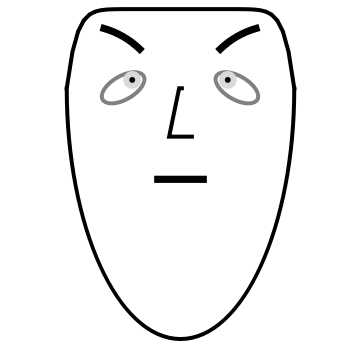The argument association can have specifications for the face part colors and face symmetry:

 In:=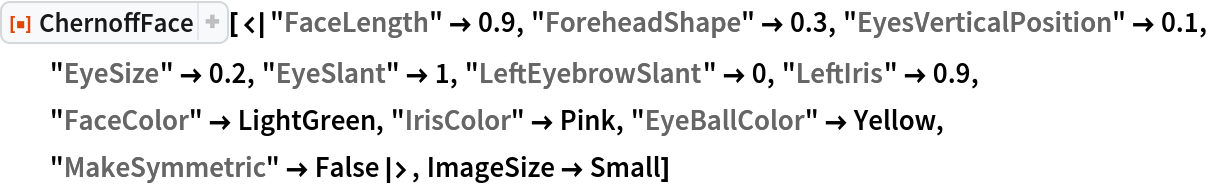Out=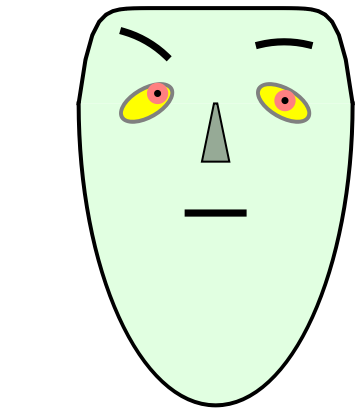The order of the face parts is based on research on which diagram features influence most influence how Chernoff face diagrams are perceived and comprehended.

#### Properties (3)

Given the argument "Properties", a default association for making Chernoff face diagrams is retrieved:

 In:=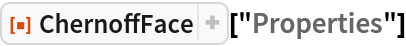Out=Use "FaceParts" or "FacePartsProperties" to get an association with only the face parts:

 In:=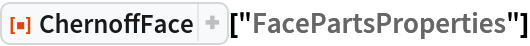Out=Here are the additional, non-numerical properties (face parts colors and symmetry):

 In:=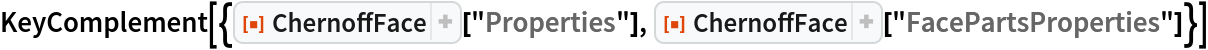Out=#### List of associations (1)

Here is a list of face-part-to-value associations:

 In:=Out=In:=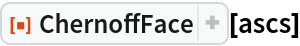Out=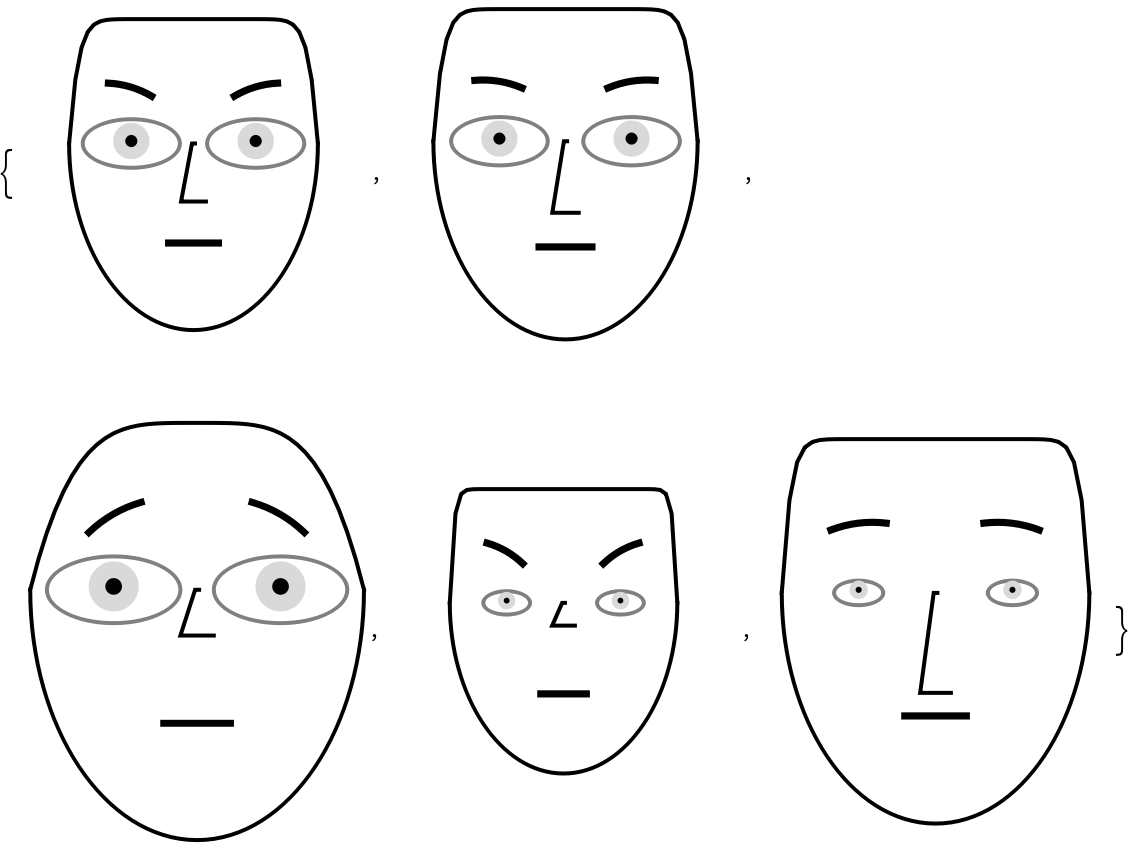### Options (9)

#### ColorFunction (2)

Using the option ColorFunction, we can produce different colorings:

 In:=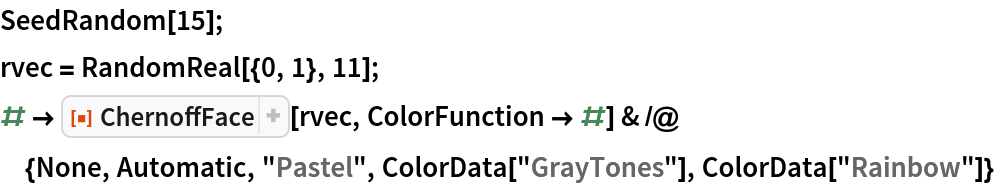Out=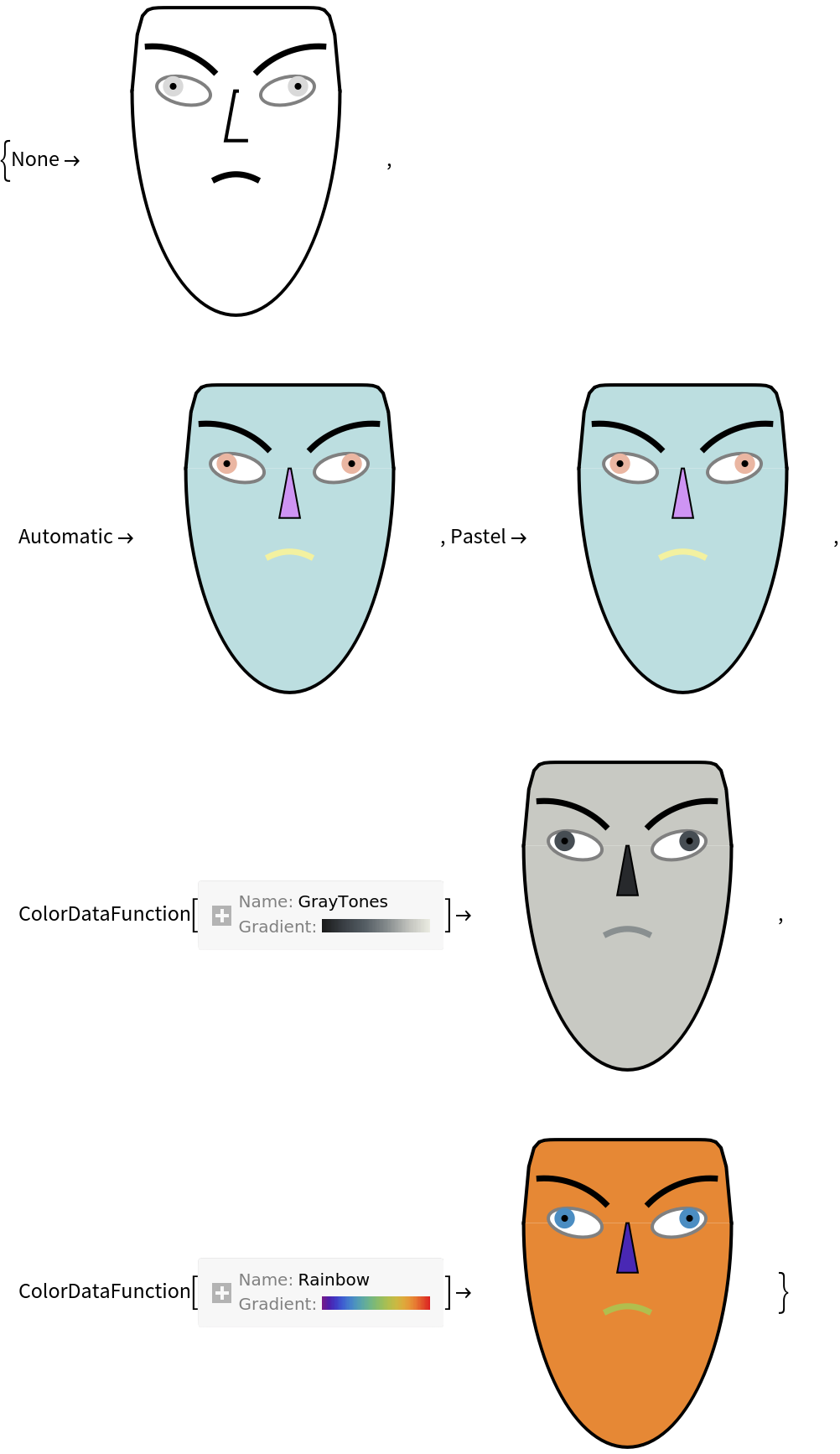The option ColorFunction assigns automatic colors to argument's association keys:

 In:=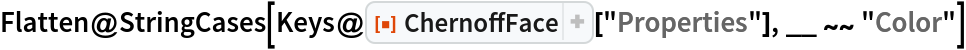Out=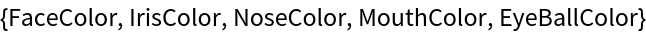#### MakeSymmetric (3)

The faces can be made symmetric for shorter records.

Here is a random numeric vector:

 In:=Here are diagrams produced with different values for the option "MakeSymmetric":

 In:=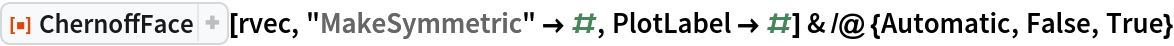Out=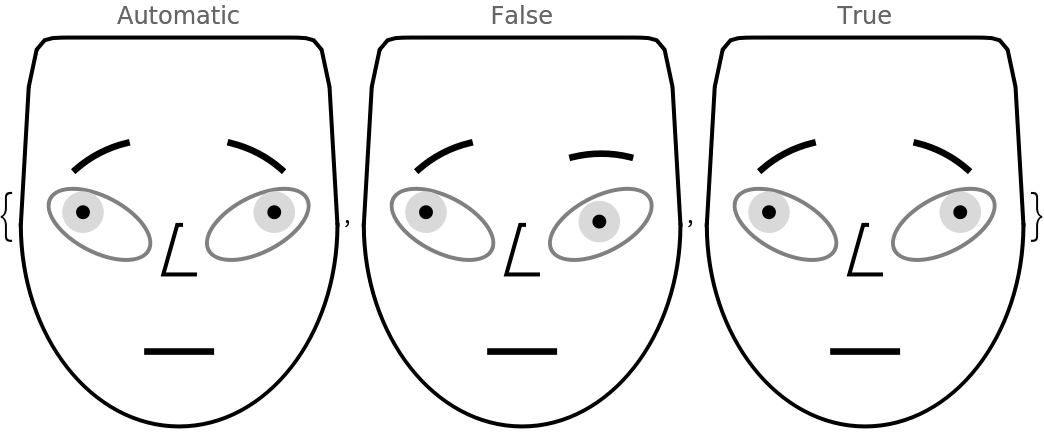The option "MakeSymmetric" overrides the argument's association value of "MakeSymmetric":

 In:=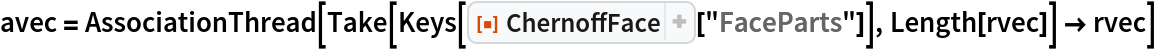Out=In:=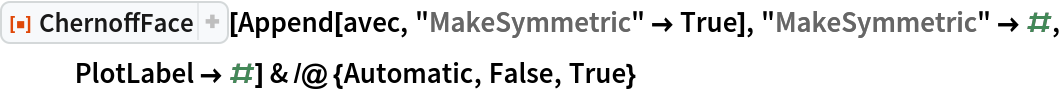Out=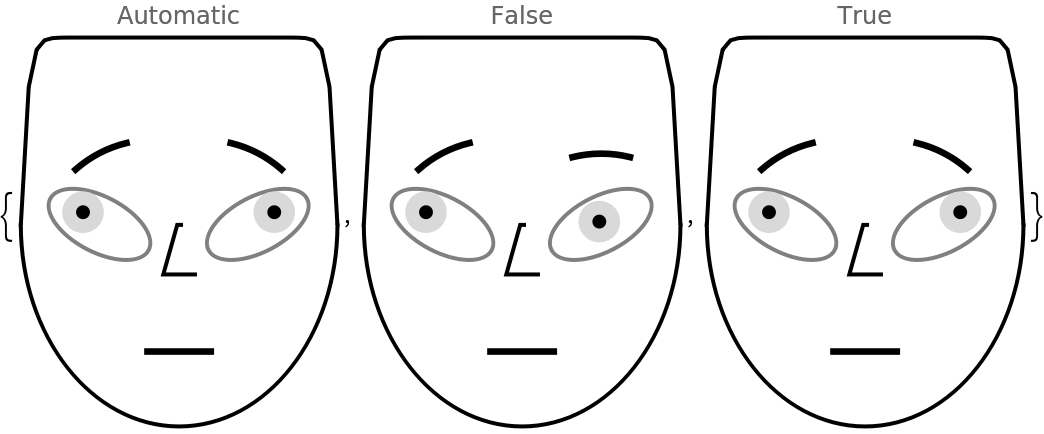#### RescaleRangeFunction (4)

If the data argument is a numeric matrix or a list of associations with numeric values, then the data columns are rescaled to run between 0 and 1. The rescaling is done with Rescale using a data-range-finding function specified as a value to the option "RescaleRangeFunction" (the default of which is MinMax).

Here is a random numerical matrix:

 In:=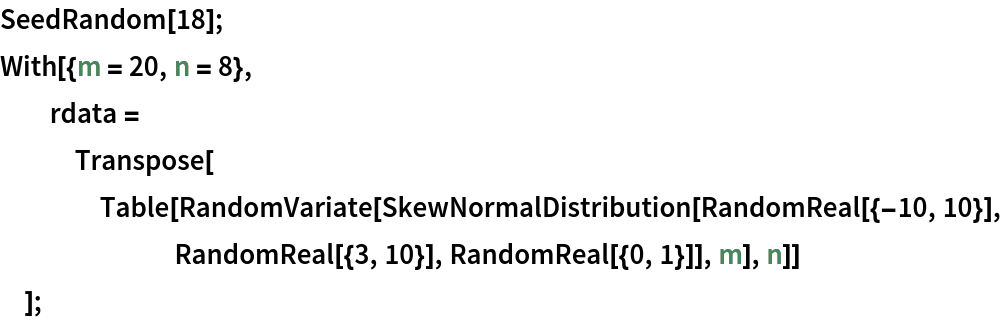Here is a summary of the matrix:

 In:=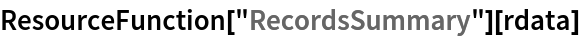Out=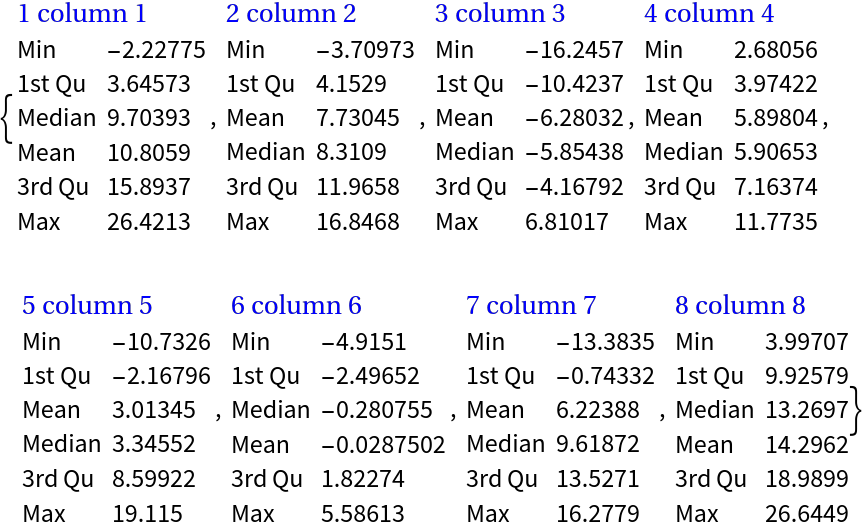Here is a list of Chernoff face diagrams produced with the default value for the option "RescaleRangeFunction":

 In:=Out=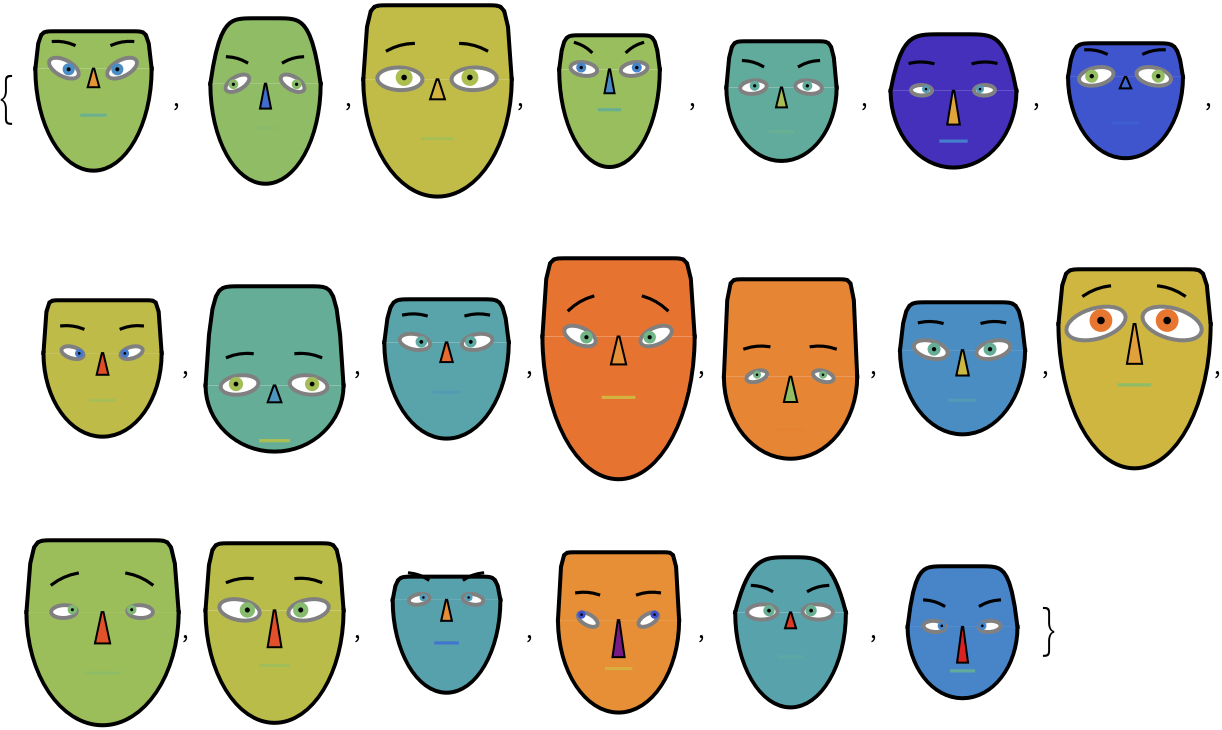Here is a list of Chernoff face diagrams produced by making the ranges of the matrix columns slightly wider than they are:

 In:=Out=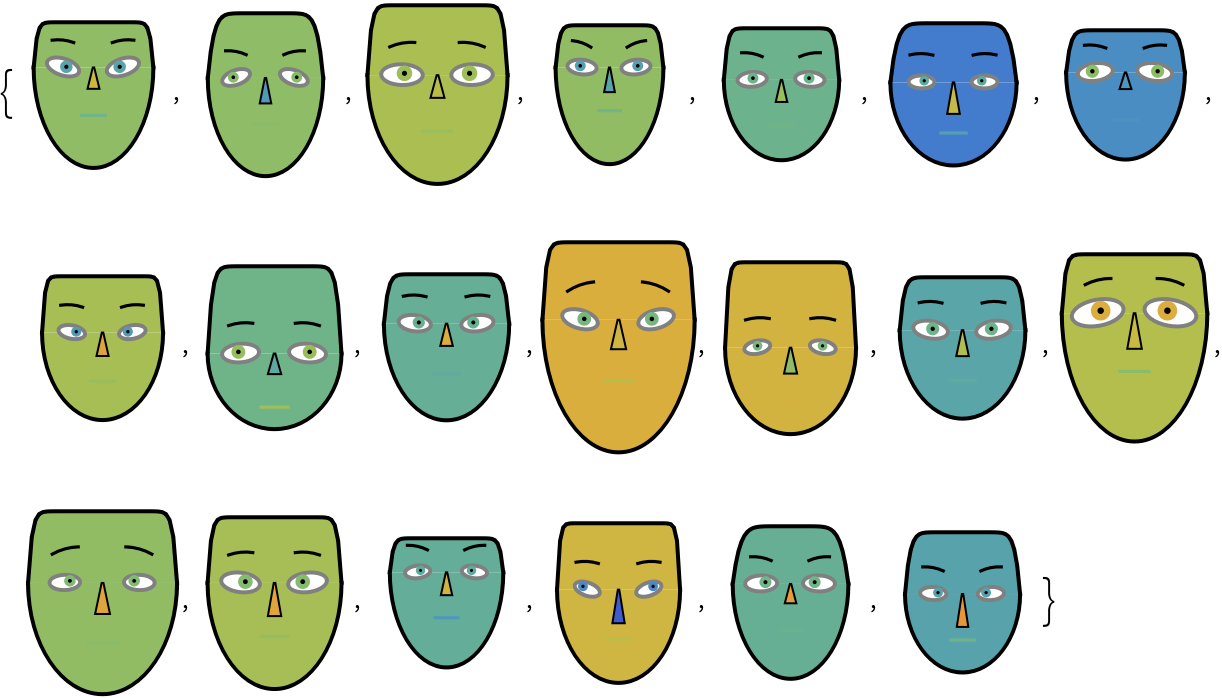### Applications (5)

#### Visualizing the "EmployeeAttitude" dataset

In order to study a dataset with Chernoff face diagrams, we have to provide: (1) a list of Chernoff face diagrams; (2) a legend that shows how the face features are mapped to dataset's columns; and (3) a legend that shows Chernoff face diagrams for easy-to-understand instances of the dataset.

Get the "EmployeeAttitude" data:

 In:=Out=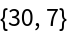Get the corresponding column names:

 In:=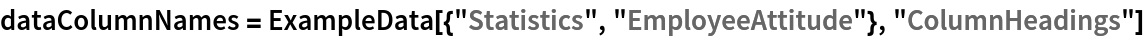Out=Here is a summary of the dataset:

 In:=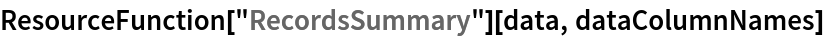Out=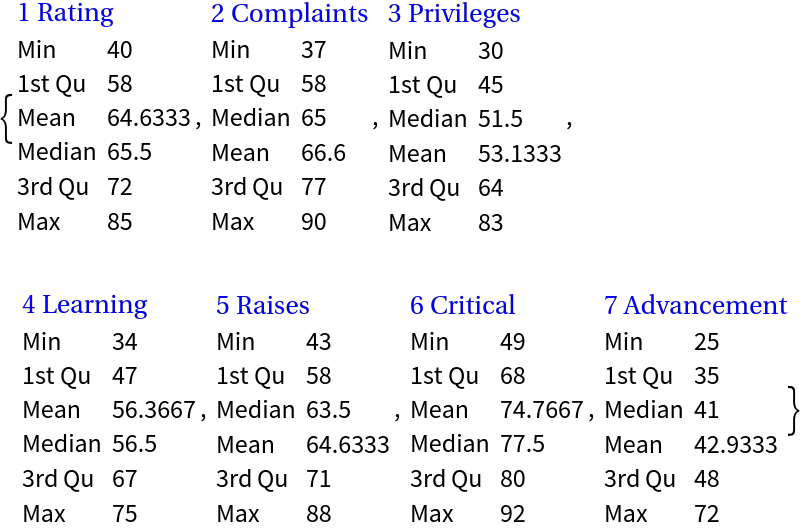Visualize with Chernoff faces:

 In:=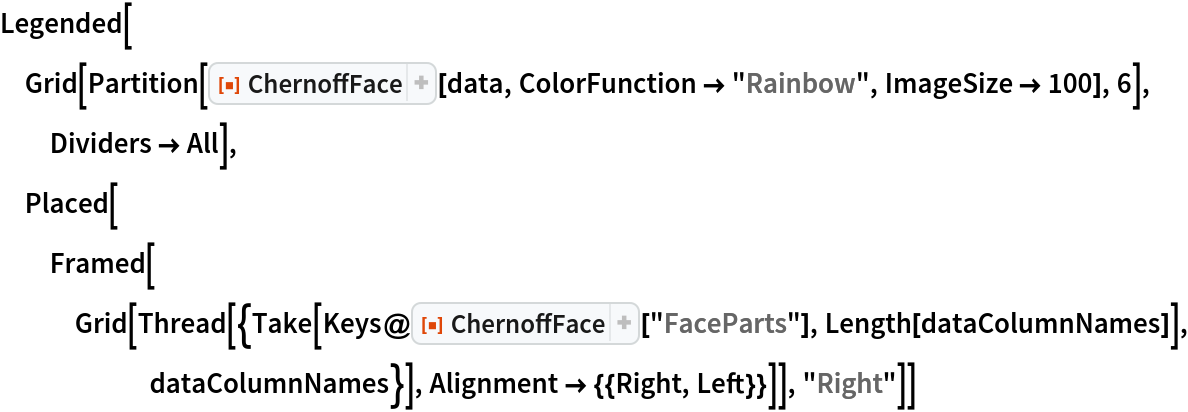Out=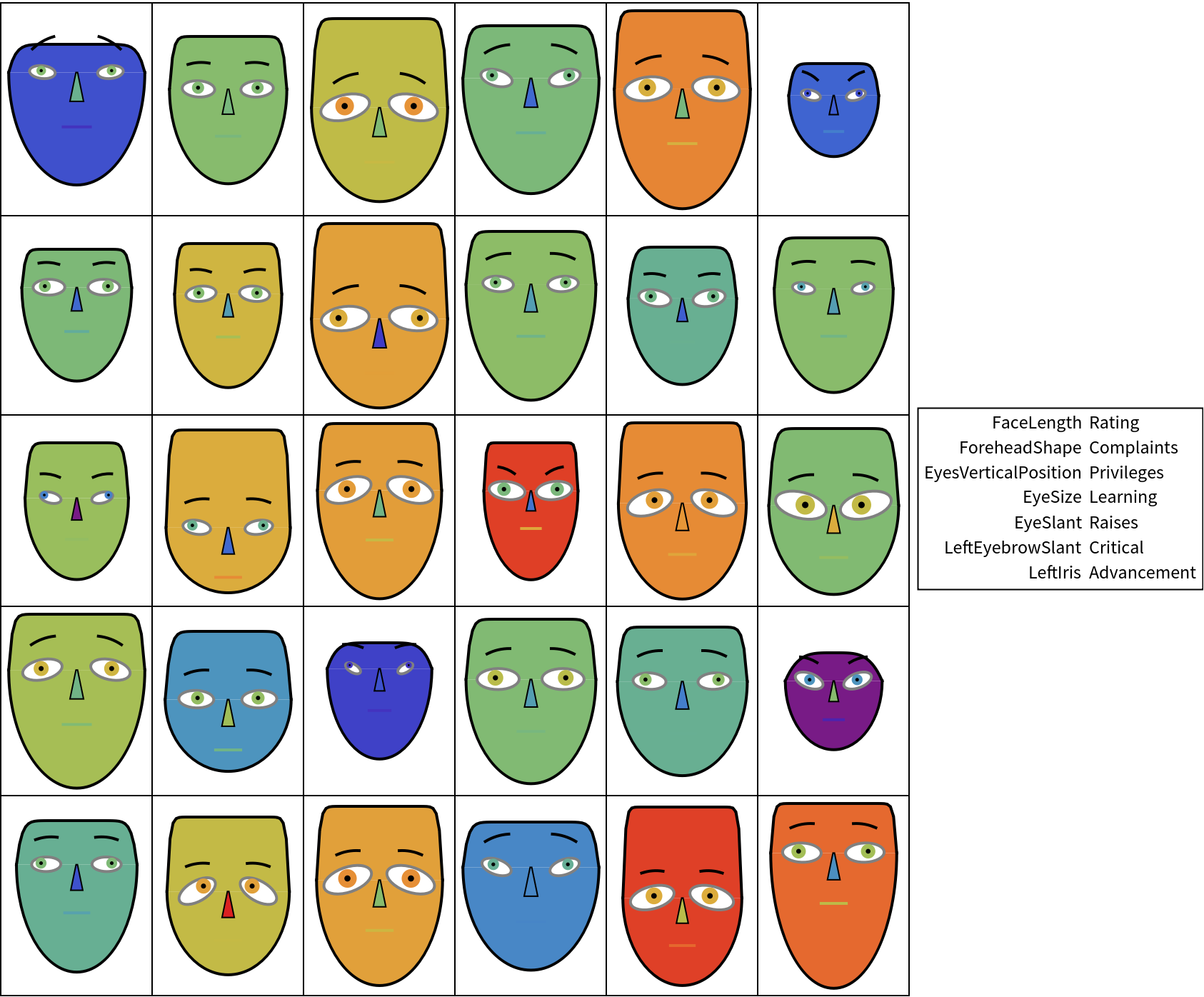Make a table of records summary diagrams:

 In:=Out=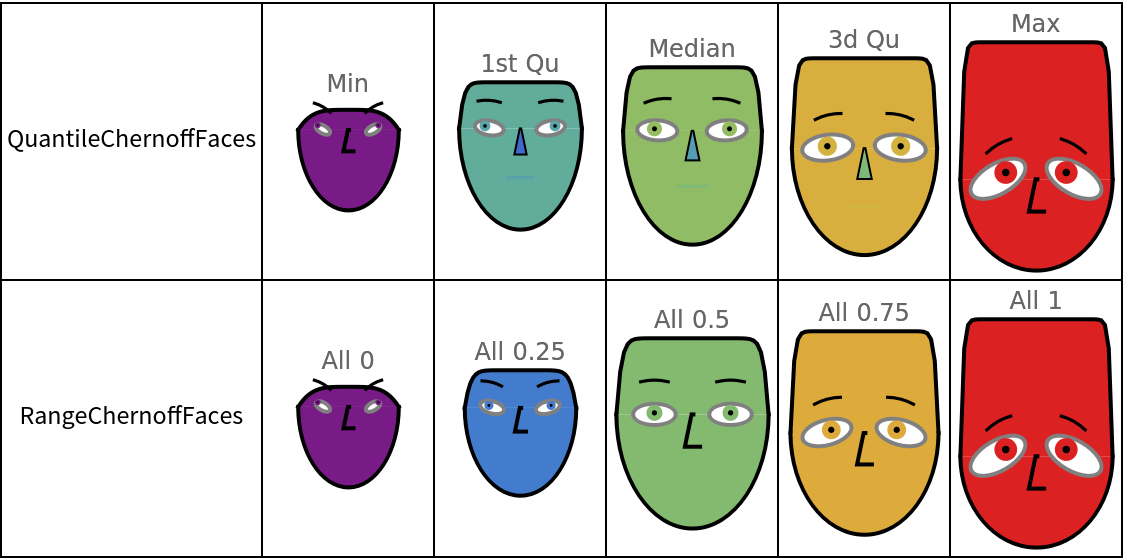We can see that: (1) higher learning is associated with higher ratings; (2) the higher the privileges, the higher the ratings. Other observations can also be made.

### Properties and Relations (1)

It is a good idea to compare the diagrams made with ChernoffFace to diagrams made with SectorChart:

 In:=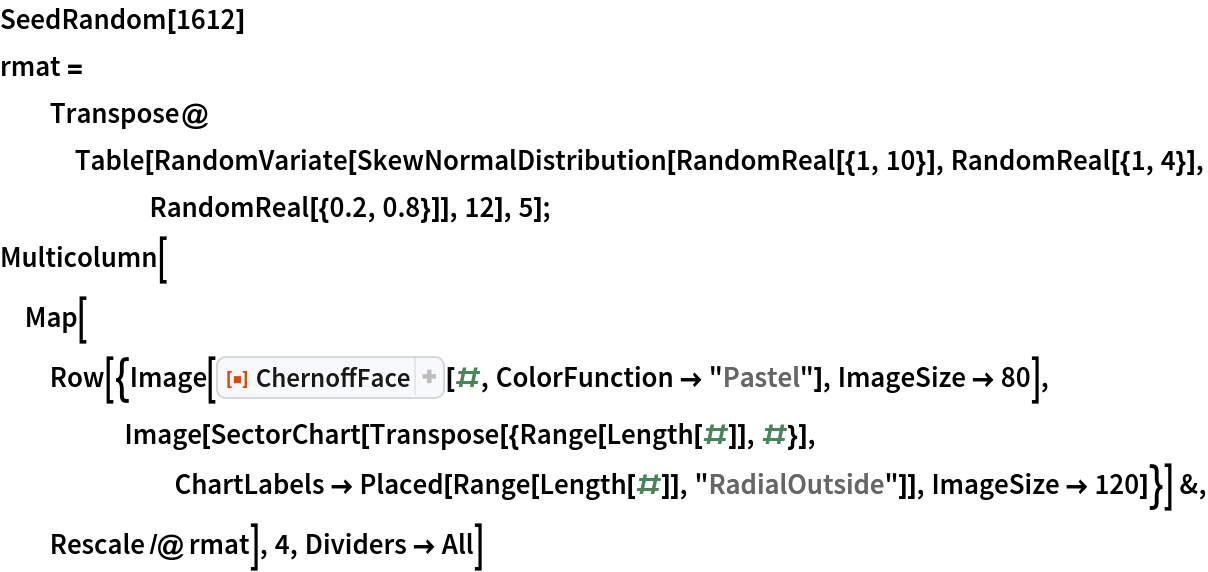Out=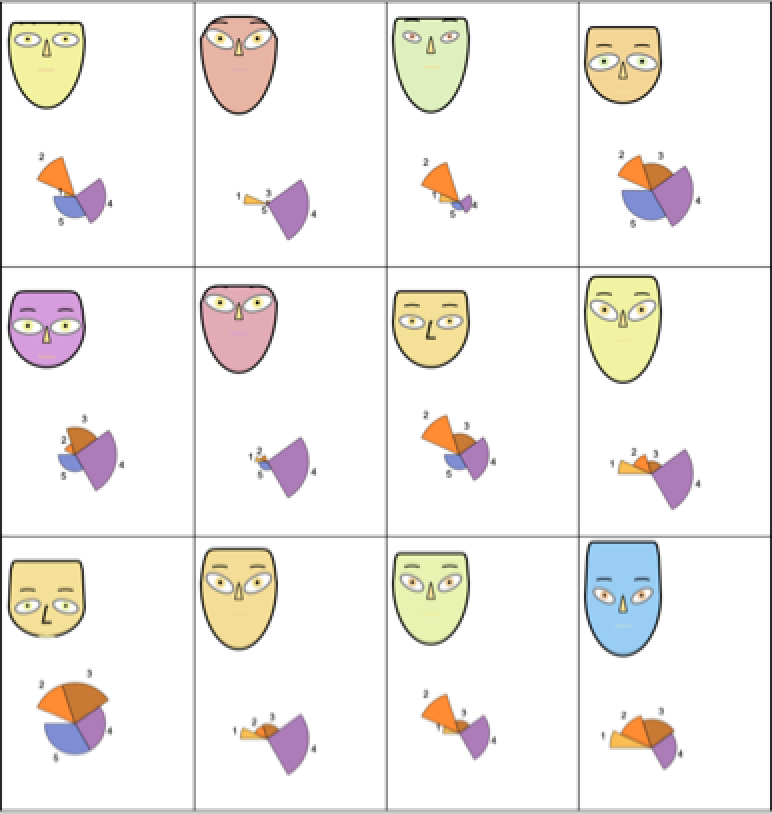### Possible Issues (5)

#### Record-wise vs. en bloc  (3)

ChernoffFace invoked over an association or a list of numbers rescales the values of the argument data to run from 0 to 1 over the range Min[data]to Max[data]. ChernoffFace called on a list of associations or a matrix rescales the columns of the corresponding full array. Hence, in general, the diagrams produced by invoking ChernoffFace record-wise over a list of records are different from the diagrams produced by invoking ChernoffFace over the whole data set of records.

Here is a random numeric matrix (list of records):

 In:=Here are Chernoff face diagrams made separately for each record:

 In:=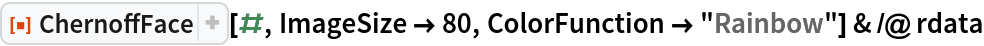Out=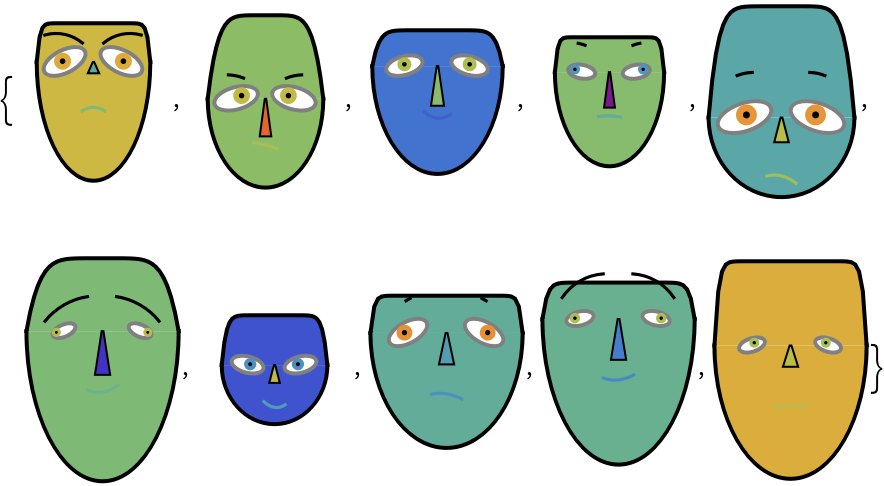Here are Chernoff face diagrams made for the whole array:

 In:=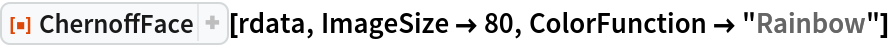Out=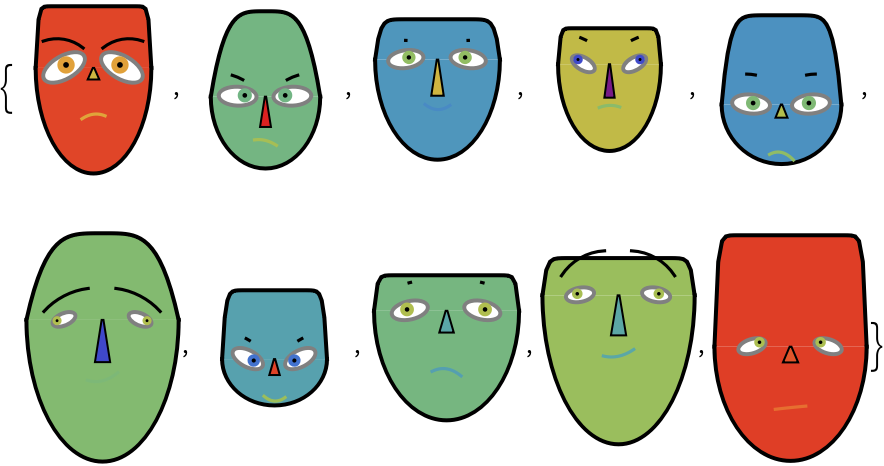We see that although the diagrams from the two lists are similar, they also have notable differences. With different data distributions, the differences will be greater or smaller.

#### Different perceptions with different color schemes (2)

The same data visualized with different color schemes might make certain items or features more obscure or more prominent.

Using the "BrightBands" color scheme makes all diagrams to look unique:

 In:=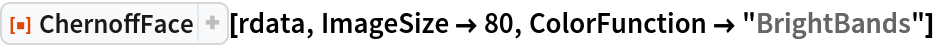Out=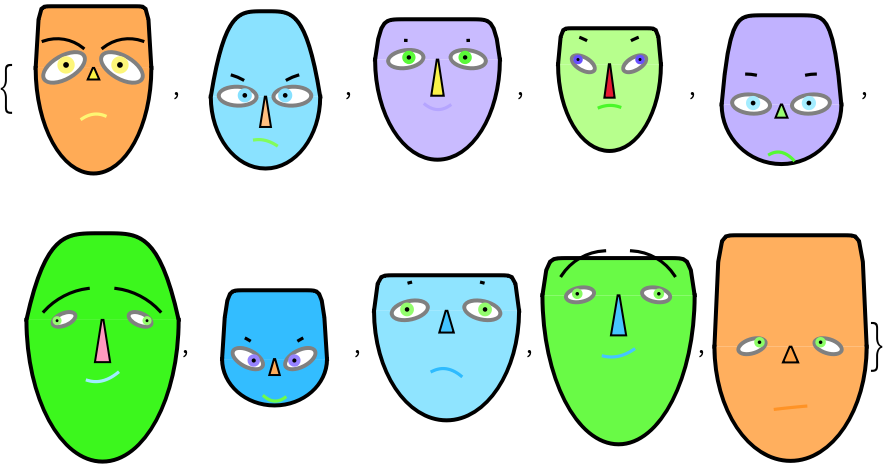Using the "WatermelonColors" color scheme over the same data makes the first and the last diagram to stand out:

 In:=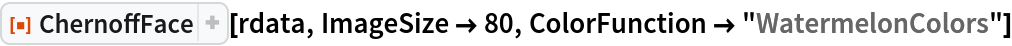Out=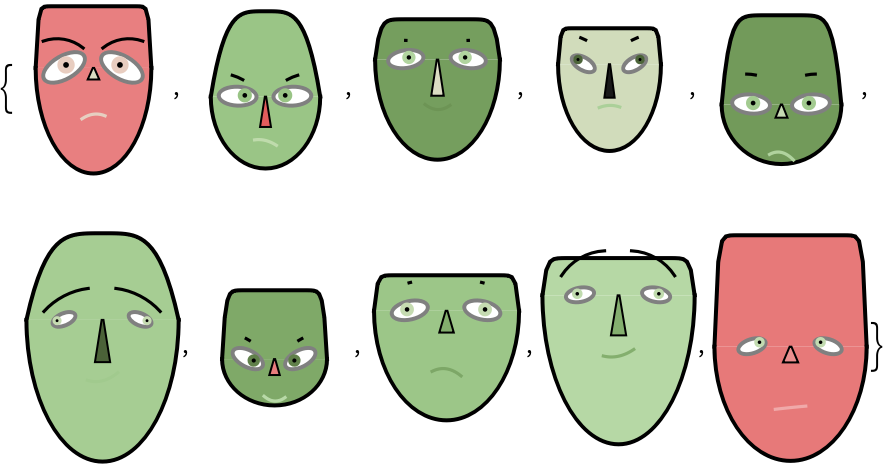### Neat Examples (1)

A table of random Chernoff faces:

 In:=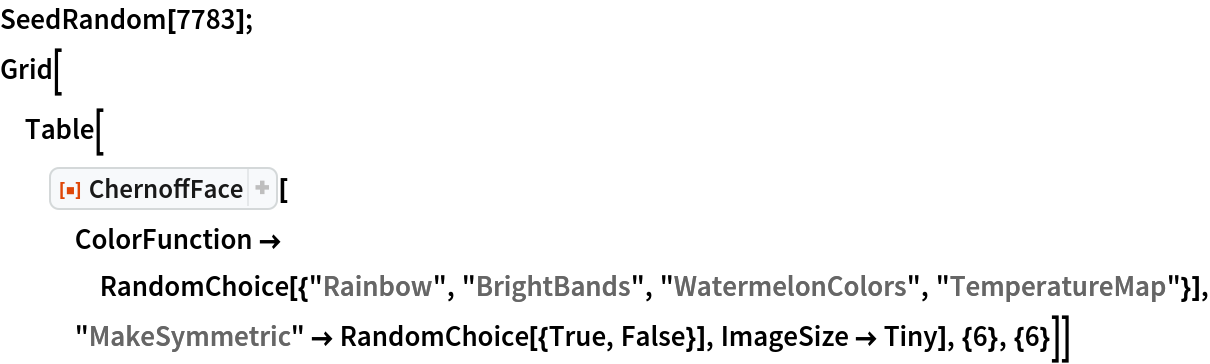Out=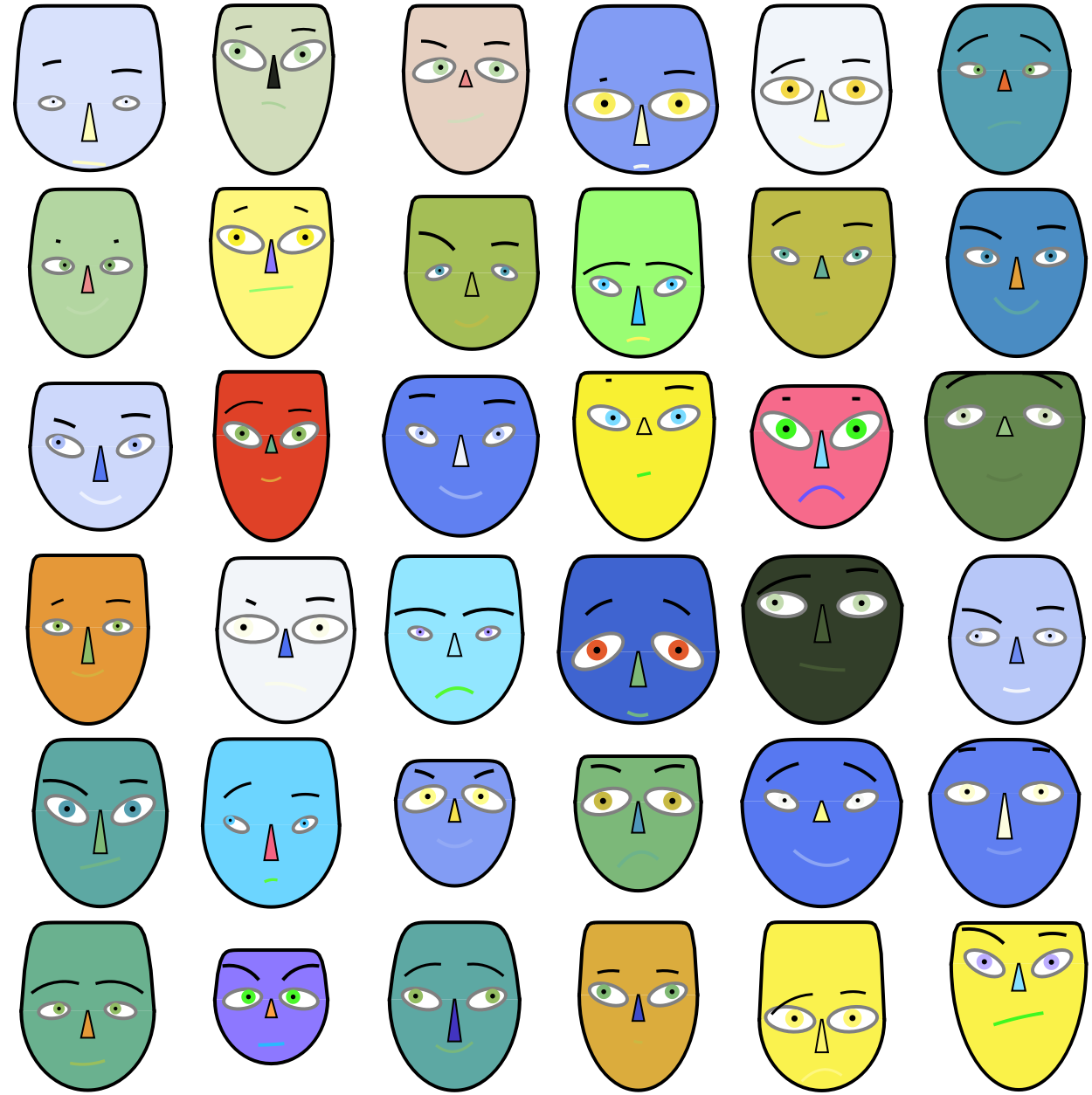## Author Notes

The idea to use human faces in order to understand, evaluate, or easily discern (the records of) multidimensional data is very creative and inspirational. I personally find the use of Chernoff face diagrams useful in a small number of cases, but that is probably true for many “creative” data visualization methods.

A fundamental restriction of using Chernoff face diagrams is the necessity to properly transform the data variables into the ranges of the Chernoff face diagram parameters. Therefore, proper data transformation (standardizing and rescaling) is an inherent part of the application of Chernoff face diagrams.

The order of the face parts is based on some research which Chernoff face diagram features influence the most diagram’s comprehension. See the article:

Christopher J. Morris; David S. Ebert; Penny L. Rheingans, “Experimental analysis of the effectiveness of features in Chernoff faces”, Proc. SPIE 3905, 28th AIPR Workshop: 3D Visualization for Data Exploration and Decision Making, (5 May 2000); doi: 10.1117/12.384865.